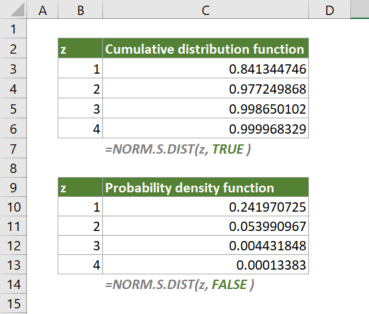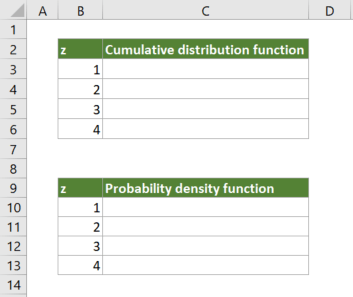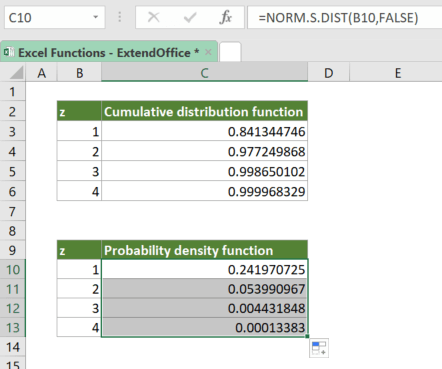## Excel NORM.S.DIST Function

The NORM.S.DIST calculates and returns the standard normal cumulative distribution function or probability density function of a value for an arithmetic mean of 0 and standard deviation of 1.#### Syntax

=NORM.S.DIST(z, cumulative)

#### Arguments

• z (required): The value for which to calculate the distribution.
• cumulative (required): A logical value that specifies the type of distribution to be used:
• TRUE: Returns the cumulative distribution function (CDF);
• FALSE: Returns the probability density function (PDF).

#### Return Value

The NORM.S.DIST function returns a numeric value.

#### Function Notes

• The #VALUE! error occurs if any of the supplied arguments is nonnumeric.

#### ExampleSuppose that you have a list of z values, to calculate the cumulative distribution function at the values, please copy or enter the formula below in top cell of the Cumulative distribution function table (cell C3), press Enter to get the first result, and then drag the fill handle of the result cell down to apply the formula to the below cells:

=NORM.S.DIST(B3,TRUE)

√ Note: The dollar signs (\$) above indicate absolute references, which means the array argument in the formula won't change when you move or copy the formula to other cells. However, there are no dollar signs added to x since you want it to be dynamic. After entering the formula, drag the fill handle down to apply the formula to the below cells.

To calculate the probability density function at the values, please copy or enter the formula below in top cell of the Probability density function table (cell 10), press Enter to get the first result, and then drag the fill handle down to apply the formula to the below cells:

=NORM.S.DIST(B10,FALSE)#### NORM.S.DIST VS. NORM.DIST

• NORM.S.DIST uses the standard normal distribution which is a special case of the normal distribution where the mean is 0 and the standard deviation is 1;
• NORM.DIST uses the normal distribution with the mean and standard deviation you specified.

#### Related functions

Excel NORM.DIST Function

The NORM.DIST calculates and returns the cumulative distribution function or probability density function of a value for the given arithmetic mean and standard deviation.

Excel NORM.INV Function

The NORM.INV calculates and returns the inverse of the normal cumulative distribution for the given arithmetic mean and standard deviation.

Excel NORM.S.INV Function

The NORM.S.INV calculates and returns the inverse of the standard normal cumulative distribution that has an arithmetic mean of 0 and standard deviation of 1 with a given probability.

### The Best Office Productivity Tools

#### Kutools for Excel - Helps You To Stand Out From Crowd

 Popular Features: Find/Highlight/Identify Duplicates   |  Delete Blank Rows   |  Combine Columns or Cells without Losing Data   |   Round without Formula ... Super Lookup: Multiple Criteria VLookup | Multiple Value VLookup |  VLookup Across Multi-Sheets |  Fuzzy Lookup... Advanced Drop-down List: Easy Drop Down List   |  Dependent Drop Down List   |  Multi-select Drop Down List .... Column Manager: Add a Specific Number of Columns   |   Move Columns   |   Unhide Columns   |   Compare Columns to Select Same & Different Cells ... Featured Features: Grid Focus   |  Design View   |   Big Formula Bar   |  Workbook & Sheet Manager   |  Resource Library (Auto Text)   |  Date Picker   |  Combine Worksheets   |  Encrypt/Decrypt Cells   |  Send Emails by List   |  Super Filter   |   Special Filter (filter bold/italic/strikethrough...) ... Top 15 Toolset:  12 Text Tools (Add Text, Remove Characters ...)   |   50+ Chart Types (Gantt Chart ...)   |   40+ Practical Formulas (Calculate age based on birthday ...)   |   19 Insertion Tools (Insert QR Code, Insert Picture from Path ...)   |   12 Conversion Tools (Numbers to Words, Currency Conversion ...)   |   7 Merge & Split Tools (Advanced Combine Rows, Split Cells ...)   |   Many More...

Kutools for Excel boasts over 300 features, ensuring that what you need is just a click away...

Supports Office/Excel 2007-2021 & newer, including 365   |   Available in 44 languages   |    Full-featured 30-day free trial.#### Office Tab - Enable Tabbed Reading and Editing in Microsoft Office (include Excel)

• One second to switch between dozens of open documents!
• Reduce hundreds of mouse clicks for you every day, say goodbye to mouse hand.
• Increases your productivity by 50% when viewing and editing multiple documents.
• Brings Efficient Tabs to Office (include Excel), Just Like Chrome, Firefox, And New Internet Explorer.